# Vector Plot

Plot vectors in space domain

• Library:
• Motor Control Blockset / Signal Management

•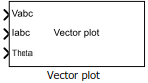## Description

The Vector Plot block plots and tracks the changes in vectors in the space domain. You can use the block to visualize vectors for electrical quantities (such as voltage and current) and track their changes in real time by using the trace left behind by the vector tip.

The block accepts vector magnitudes and their angles (in radians, per-unit, or degrees) as inputs and provides a pictorial representation of the vectors. The block also traces the plot history of the vector tip according to the selected number of points.

For details about how to use the Vector Plot block, see the model `mcb_pmsm_foc_qep_f28379d` in Field-Oriented Control of PMSM Using Quadrature Encoder.

## Ports

### Input

expand all

Voltage components in the three-phase system in the abc reference frame. The port accepts three voltage components multiplexed by using Mux.

#### Dependencies

To enable this port, set Select input types to `Vabc Iabc Theta`.

Data Types: `single` | `double`

Current components in the three-phase system in the abc reference frame. The port accepts three current components multiplexed by using Mux.

#### Dependencies

To enable this port, set Select input types to `Vabc Iabc Theta`.

Data Types: `single` | `double`

Angle value (in radians, per-unit, or degrees for `Vabc` and `Iabc`) between the rotating reference frame and the α-axis.

The figures show the angle of transformation when:

• The d-axis aligns with the α-axis.• The q-axis aligns with the α-axis.In both cases, the angle is Theta = ωt, where:

• Theta is the angle between the α- and d-axes for the d-axis alignment or the angle between the α- and q-axes for the q-axis alignment. It indicates the angular position of the rotating dq reference frame with respect to the α-axis.

• ω is the rotational speed in the d-q reference frame.

• t is the time in seconds from the initial alignment.

#### Dependencies

To enable this port, set Select input types to `Vabc Iabc Theta` and set Select reference frame to `Rotating Reference Frame`.

Data Types: `single` | `double`

Direct and quadrature axis voltage components in the rotating dq reference frame. The port accepts two voltage components multiplexed by using Mux.

#### Dependencies

To enable this port, set Select input types to `Vdq Idq`.

Data Types: `single` | `double`

Direct and quadrature axis current components in the rotating dq reference frame. The port accepts two currents components multiplexed by using Mux.

#### Dependencies

To enable this port, set Select input types to `Vdq Idq`.

Data Types: `single` | `double`

Magnitudes of vectors that you want to plot. The port accepts up to six vector magnitudes multiplexed by using Mux. The vector magnitudes correspond to the angle values input to the Angle port.

Note

The number of multiplexed vector magnitudes should be same as the number of multiplexed angles input to the Angle port.

#### Dependencies

To enable this port, set Select input types to `Mag Angle`.

Data Types: `single` | `double`

Angle values (in radians, per-unit, or degrees) of vectors that you want to plot. The port accepts up to six vector angles multiplexed by using Mux. The angle values correspond to the vector magnitudes input to the Magnitude port.

Note

The number of multiplexed vector angles should be same as the number of multiplexed magnitudes input to the Magnitude port.

#### Dependencies

To enable this port, set Select input types to `Mag Angle`.

Data Types: `single` | `double`

## Parameters

expand all

Types of input ports available for the block.

Select type of reference frame that the block uses to plot the input vectors:

• `Rotating Reference Frame` — Select this option to plot the three-phase voltage and current vectors in the rotating dq reference frame.• `Stationary Reference Frame` — Select this option to plot the three-phase voltage and current vectors in the stationary αβ reference frame.#### Dependencies

To enable this parameter, set Select input types to `Vabc Iabc Theta`.

Align either the d- or q-axis of the rotating reference frame to the α-axis of the stationary reference frame.

#### Dependencies

To enable this parameter, set Select input types to `Vabc Iabc Theta` and Select reference frame to `Rotating Reference Frame`.

Unit of Theta input value.

#### Dependencies

To enable this parameter, set Select input types to `Vabc Iabc Theta` and Select reference frame to `Rotating Reference Frame`.

Unit of Angle input value.

#### Dependencies

To enable this parameter, set Select input types to `Mag Angle`.

Select this parameter to automatically open the vector plot window when simulation begins.

— Click this button to open the vector plot window.

### Vector Plot Window

This example shows the plot when you set Select input types to `Mag Angle` and provide three multiplexed magnitudes and three multiplexed angles as inputs.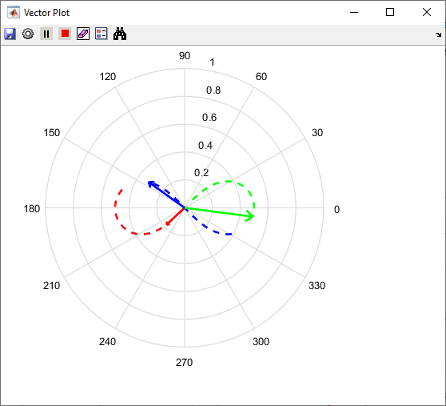You can use these buttons on the Vector Plot window:

•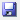(Save Figure) — Click to save the plot to an image.

•(Preferences) — Click to open the Preferences dialog box.

• Display traces (samples) — Enter the number of samples that you want to trace for the vector tip. By default, the field uses the value `100`.

• Auto-Scale — Select this field to automatically scale the axes limit. The block performs auto-scaling every at every `1000` points of simulation.

• Axes limit — Enter the maximum value of the x and y axis that the plot should use. By default, the field uses the value `1`. If you select Auto-Scale and the vector magnitude increases beyond the selected axes limit, the limit on the Vector Plot window extends automatically to accommodate the vector magnitude.

•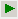(Run Simulation) — Click to simulate the model that contains the Vector Plot block.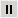(Pause Simulation) — Click to pause simulation.

•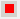(Stop Simulation) — Click to stop simulation.

•(Clear Data History) — Click to clear the vector tracing history.

•(Insert Legend) — Click to insert or remove the legend describing the vectors. You can manually change the default legend description.•(Auto Scale) — Click to turn on or turn off the auto-scale function.